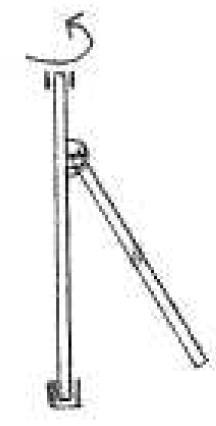# Solving Swinging Pendulum Homework

• abotiz

## Homework Statement

A pendulum is mounted on a vertical, rotating
shaft by means of a hinge. The shaft is free
to rotate in its bearings. Use conservation laws to
eliminate one degree of freedom and simulate the
motion of the other one. Analyse and comment
using mechanical terms.

https://www.physicsforums.com/attachment.php?attachmentid=18687&d=1241008388

## The Attempt at a Solution

I started out with the Energy principle: E = constant = Kinetic + Potential
U = Potential------I = Moment of inertia
K= Kinectic------w = angular velocity
m = mass------v = velocity
L = Length------ θ = Angle

K = ½(*I*w2+*m*v2)

U = -½*m*g*L*cos θ

And i have no idea what to do next, i think i perhaps should derive the energy expression, but i don't know what to derive on, time,angle?

Thank you!

Sorry, problem with image

Here it is